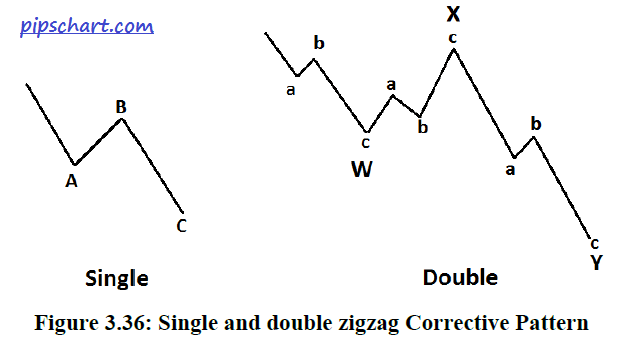# Corrective Waves the 2nd part of waves

1,270 views

Corrective waves are unquestionably the most difficult to interpret. They can appear in many different configurations and pattern combinations. Richard Swannell and Robert Prechter have both contributed to our current understanding of corrective waves. Swannell has tested price data, searching for waves, and Prechter has interpreted Elliott’s original work with some modifications.

The following information about corrective waves comes from the work of these two analysts. Corrective waves commonly occur in three sub waves (A-B-C). The direction of corrective waves is either sideways, as in a trading range, or sharply opposite to the prevailing trend of the impulse wave. Corrective waves are generalized into three categories for descriptive purposes: zigzags, flats, and triangles.

Zigzags
The single A-B-C correction in Figure 3.36 is an example of a zigzag correction. Generally, in a simple zigzag, wave A is an impulse, leading diagonal, or zigzag; wave B is any corrective pattern; and wave C is an impulse, ending diagonal, or zigzag. If wave A is a diagonal, wave C is not an ending diagonal.

In addition to this simple, single A-B-C correction, a zigzag correction can occur as a more complex double or triple zigzag pattern. The second correction pictured in Figure 3.36 shows the more complex double zigzag. Double and triple zigzags use different nomenclature for their descriptions. The double zigzag, for example, is two simple zigzags joined by a wave X.As shown in Figure 3.36, W and Y are often used to designate each simple zigzag wave within the double zigzag. When another simple zigzag is added to a double zigzag, a triple zigzag forms. The added simple zigzag is referred to as wave Z; the intervening wave is labeled XX. Fortunately, for interpretive purposes, triple zigzags are rare.

Swannell (2003) provides the following characteristics for actual double zigzags:
• Wave W is a zigzag
• Wave X is any corrective pattern other than an expanding triangle
• Wave X is smaller than wave W
• Wave Y is a zigzag
• Wave Y is equal to or larger than wave X

Triple zigzags continue with the same pattern as double zigzags; thus, wave XX is smaller than both wave Y and Z. Prechter points out that zigzags characteristically have a 5-3-5 combination of sub waves.

Flats
Sideways patterns are called flats. Flats behave very similarly to zigzags except that rather than sharp upward or downward corrections to the prevailing trend, they move sideways, and their sub waves generally overlap. The characteristics for flats are the same as for zigzags except that

• Wave B is not a triangle, retraces wave A by at least 50%, and is less than twice the length of wave A.
• Wave C overlaps wave A at some point.

The A-B-C pattern in Figure 3.37 shows a simple flat pattern. In addition to this single A-B-C pattern, flats can occur as a double or a triple. Double and triple sideways have the same nomenclature as double and triple zigzags. Just like triple zigzags, triple sideways are rare.The characteristics of the double and triple flats are similar to their zigzag counterparts except for the necessary adjustments needed to produce a sideways pattern. Thus, common characteristics of these multiple flat patterns are as follows:

• Waves W, X, and XX can be any corrective pattern except a triangle, a double, or a triple.
• Waves Y and Z can be any corrective pattern except a double or triple.
• Wave X retraces at least 50% of wave W.
• Wave Y is greater than wave X unless it is a triangle.
• Wave XX retraces at least 50% of wave Y.

• Wave Z is not a zigzag if wave Y is a zigzag.
• Wave Z is greater than wave XX.

Prechter points out that flats characteristically have a 3-3-5 combination of sub waves. Elliott, he argues, considered the sideways patterns as possibly including a combination of zigzag and flat, one followed by the other in a complex corrective wave. These were called doubles and triple threes. The method of designating each pattern is the same as the method used
previously for double and triple sideways patterns.

Triangles
The Elliott wave triangle is identical to the standard triangle pattern, “Bar Chart Patterns,” with the exception that it is limited to five waves (A-B-C-D-E). Prechter points out that triangles have a 3-3-3-3-3 combination of minor waves. The Elliott wave triangle can be either a converging or a diverging triangle.

The rules for a converging triangle are as follows:
• Wave A is a zigzag or a flat.
• Wave B is only a zigzag.
• Waves C and D can be any corrective pattern except a triangle.
• Waves A, B, C, and D move within the bounds of the channel lines between A to C and B to D.
• Bounds converge (one may be horizontal), and the intersection of the bounds occurs beyond the end of wave E.
• Wave E can be a zigzag or a converging triangle.
• Either wave A or B is the longest wave.
• Wave E ends in the range of wave A.
• Wave E moves within or closes within the bounds. Expanding triangles, although relatively rare, have generally the same rules. The exceptions are that for the expanding triangle:
• Wave B is smaller than wave C but more than 40% of wave C.
• Wave C is smaller than wave D but more than 40% of wave D.
• The intersection of the bounds occurs before the formation of the triangle.
• Wave E is longer than wave D.

• Wave E ends outside the territory of wave A.
• Either wave A or B is the shortest wave.